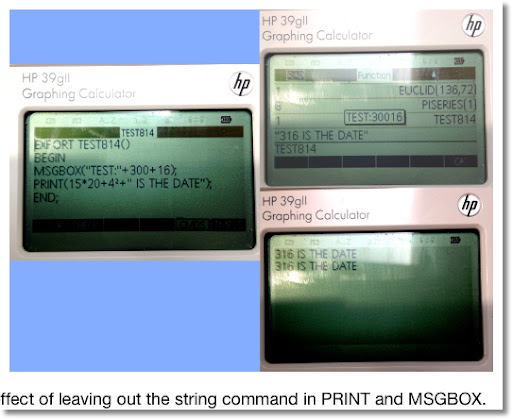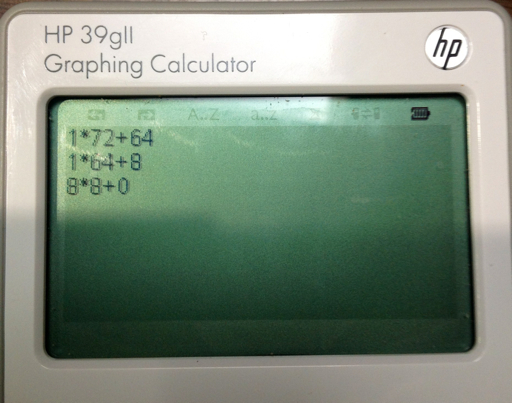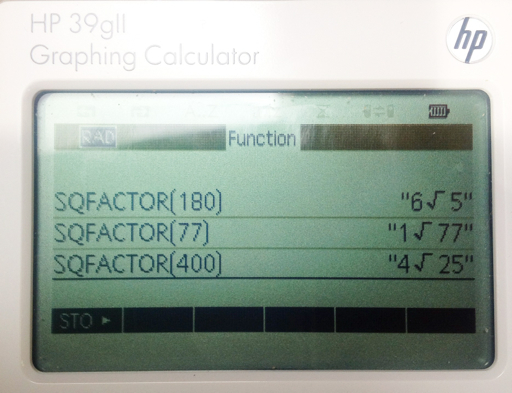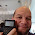## Monday, March 18, 2013

### HP 39gii Programming Part 4: REPEAT and WHILE

Welcome to Part 4 of the HP 39gii Programming Tutorial. Here is what is on the menu tonight:

1. REPEAT structure
2. WHILE structure
3. Some Notes
4. The Program EUCLID
5. The Program RSUM1
6. The Program SQFACTOR

This lesson covers the REPEAT and WHILE loops. Each loop repeats a set of instructions indefinitely until a condition is met.

1. The REPEAT Structure

REPEAT
these instructions;
UNTIL this condition is true;

The REPEAT loop executes the set of commands then it tests the condition. Hence in a REPEAT loop, the commands are always executed at least once.

Keystrokes to find the template: In the program editor press [ F6 ] (TMPLT) [ 4 ] [ 4 ]

2. The WHILE Structure

WHILE this condition is true DO
these instructions;
END;

The WHILE loop tests the condition before executing the set of commands. It is possible that the commands in a WHILE loop are never executed.

Keystrokes to find the template: In the program editor press [ F6 ] (TMPLT) [ 4 ] [ 3 ]

3. Some Notes

Loops (FOR, WHILE, REPEAT) can be nested That is, loops can contain other loops. The is demonstrated in the SQFACTOR program, and will be demonstrated in future programs.

PRINT does not need the string command - expressions will evaluate automatically. This will allow to combine strings and mathematical expressions quickly with just the use of the plus sign (+) and the quotation marks (" "). MSGBOX makes everything in its argument a string, so it is best to use the string command. The program listed below demonstrates the difference.

EXPORT TEST814()
BEGIN
MSGBOX("TEST" + 300 + 16);
PRINT(15*20+4² + " IS THE DATE");
END;4. The Program EUCLID

The goal of EUCLID is to use the Euclidean algorithm to find the greatest common divisor of two integers. The integers can be entered in any order. EUCLID takes the two integers as pass-through arguments.

The program EUCLID
EXPORT EUCLID(X,Y)
BEGIN
LOCAL M,N,R,A;
PRINT();
N:=MAX(X,Y);
M:=MIN(X,Y);
REPEAT
A:=INT(N/M);
R:=N - A*M;
PRINT(A + "*" + M + "+" + R);
WAIT(1);
N:=M;
M:=R;
UNTIL R==0;
RETURN N;
END;

The integer is split into its integer of the quotient, A, and remainder, R, as such:

N = A * M + R

The program repeats until R=0.

Examples:
EUCLID(145,54) → 1
EUCLID(136,72) → 8 (we have a picture of this example)5. The Program RSUM1

The program RSUM1 builds a sum of random numbers between 0 and 1 while the total sum is less than 1. RSUM1 takes no arguments.

The Program RSUM1

EXPORT RSUM1()
BEGIN
LOCAL S;
S:=0;
WHILE S<1 DO
S:=S+RANDOM;
END;
RETURN S;
END;

You can find the RANDOM command in the Math-Probability menu ([Math] [Math] [ 4 ]). To generate random numbers between 0 and 1, either RANDOM or RANDOM() is acceptable.

Example sums you may get with RSUM1 are 1.29441419992, 1.28901948188, and 1.57880668903.

6. The program SQFACTOR

The program SQFACTOR simplifies √N where N is an integer (i.e. √180 = 6 √5, √364 = 2 √91). It has one pass-through argument, N.

I am going to use the store arrow (STO>) accessed by the [ F1 ] key in the program editor. The store arrow is a thick dark arrow pointing to the right (▸). You can use the := assignment if you wish.

SQFACTOR(N)
EXPORT SQFACTOR(N)
BEGIN
LOCAL C,K;
1 ▸ C;
2 ▸ K;
WHILE K² < N DO
WHILE FRAC(N/K²) == 0 DO
N/K² ▸ N;
C*K ▸ C;
END;
K+1 ▸ K;
END;
RETURN string(C)+"√"+string(N);
END;

Examples:
SQFACTOR(180) returns "6 √ 5"
SQFACTOR(77) returns "1 √ 77"
SQFACTOR(400) returns "4 √ 25"

OK The last one should be 20. However 4 √25 = 4 × 5 = 20. So this isn't perfect.

Challenge: can this be improved? I will leave this for the readers.That is it for Part 4. Next time, we will cover basic graphics commands. Take care and have fun!

Eddie

This blog is property of Edward Shore. 2013

1.2."OK The last one should be 20. However 4 √25 = 4 × 5 = 20. So this isn't perfect.

Challenge: can this be improved? I will leave this for the readers. "

it could be done : just reverse the loop :
...
K:=FLOOR(√N);
...
K:=K-1;
...

SQFACTOR(400) -> 20.√1

and modify to dont display special case √1

3.Very good Giles! Thanks for the suggestion.

Eddie